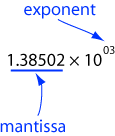Yes. But before a floating point standard this was sometimes not true.

# Scientific Notation

To address this problem the IEEE (Institute of Electrical and Electronics Engineers) created a standard for floating point. This is the IEEE 754 standard, released in 1985 after many years of development. All hardware and software since then follow this standard (many processors also support older standards as well).

The idea of floating point comes from scientific notation for numbers. You have probably seen this before (and may already be shuddering). Here is a number in scientific notation:The mantissa always has a decimal point after the first digit. The above expression means:

```1.38502 × 1000 = 1385.02
```

The decimal point "floats" to where it belongs. In effect, the mantissa gives the digits and the exponent says where the decimal point should go.

### QUESTION 6:

Write the following in scientific notation: `243.92`